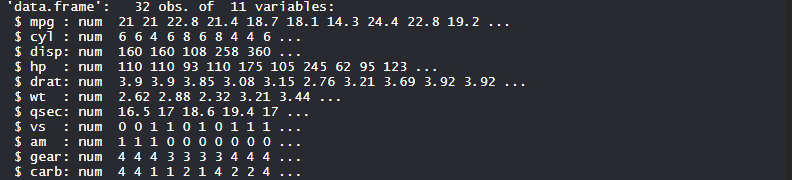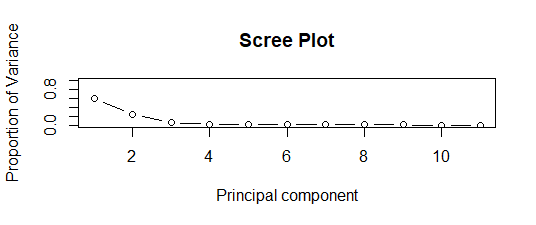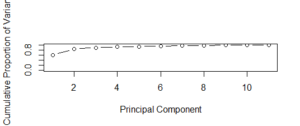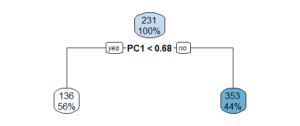# Principal Component Analysis with R Programming

Principal component analysis(PCA) in R programming is analysis on the linear components of all existing attributes. Principal components are linear combination(orthogonal transformation) of the original predictor in the dataset. It is a useful technique for EDA(Exploratory data analysis) and allowing you to better visualize the variations present in a dataset with many variables.

First principal component captures the maximum variance in dataset. It determines direction in of higher variability. Second principal component captures the remaining variance in data and is uncorrelated with PC1. The correlation between PC1 and PC2 should be zero. So, all succeeding principal components follows the same concept. They capture the remaining variance without being correlated to previous principal component.

#### The Dataset

The dataset `mtcars`(motor trend car road test) comprises fuel consumption and 10 aspects of automobile design and performance for 32 automobiles. It comes pre-installed with dplyr package in R.

 `# Installing required package ` `install.packages(``"dplyr"``) ` ` `  `# Loading the package ` `library(dplyr) ` ` `  `# Importing excel file ` `str``(mtcars) `#### Performing PCA using dataset

We perform Principal component analysis on `mtcars` which consists of 32 car brands and 10 variables.

 `# Loading Data ` `data(mtcars) ` `  `  `# Apply PCA using prcomp function ` `# Need to scale / Normalize as  ` `# PCA depends on distance measure ` `my_pca <``-` `prcomp(mtcars, scale ``=` `TRUE,  ` `                 ``center ``=` `TRUE, retx ``=` `T) ` `names(my_pca) ` `  `  `# Summary ` `summary(my_pca) ` `my_pca ` `  `  `# View the principal component loading ` `# my_pca\$rotation[1:5, 1:4] ` `my_pca\$rotation ` `  `  `# See the principal components ` `dim(my_pca\$x) ` `my_pca\$x ` `  `  `# Plotting the resultant principal components ` `# The parameter scale = 0 ensures that arrows ` `# are scaled to represent the loadings  ` `biplot(my_pca, main ``=` `"Biplot"``, scale ``=` `0``) ` `  `  `# Compute standard deviation ` `my_pca\$sdev ` `  `  `# Compute variance ` `my_pca.var <``-` `my_pca\$sdev ^ ``2` `my_pca.var ` `  `  `# Proportion of variance for a scree plot ` `propve <``-` `my_pca.var ``/` `sum``(my_pca.var) ` `propve ` `  `  `# Plot variance explained for each principal component ` `plot(propve, xlab ``=` `"principal component"``, ` `             ``ylab ``=` `"Proportion of Variance Explained"``, ` `             ``ylim ``=` `c(``0``, ``1``), ``type` `=` `"b"``,  ` `             ``main ``=` `"Scree Plot"``) ` `  `  `# Plot the cumulative proportion of variance explained ` `plot(cumsum(propve),  ` `     ``xlab ``=` `"Principal Component"``, ` `     ``ylab ``=` `"Cumulative Proportion of Variance Explained"``, ` `     ``ylim ``=` `c(``0``, ``1``), ``type` `=` `"b"``) ` `  `  `# Find Top n principal component  ` `# which will atleast cover 90 % variance of dimension ` `which(cumsum(propve) >``=` `0.9``)[``1``] ` `  `  `# Predict mpg using first 4 new Principal Components ` `# Add a training set with principal components ` `train.data <``-` `data.frame(disp ``=` `mtcars\$disp, my_pca\$x[, ``1``:``4``]) ` `  `  `# Running a Decision tree algporithm ` `## Installing and loading packages ` `install.packages(``"rpart"``) ` `install.packages(``"rpart.plot"``) ` `library(rpart) ` `library(rpart.plot) ` `  `  `rpart.model <``-` `rpart(disp ~ .,  ` `                     ``data ``=` `train.data, method ``=` `"anova"``) ` `  `  `rpart.plot(rpart.model) `

Output:

• Bi plotThe resultant principal components are plotted as Biplot. Scale value 0 represents that arrows are scaled representing loadings.

• Variance explained for each principal componentScree Plot represents the proportion of variance and principal component. Below 2 principal components, there is maximum proportion of variance as clearly seen in the plot.
• Cumulative proportion of varianceScree Plot represents the Cumulative proportion of variance and principal component. Above 2 principal components, there is maximum cumulative proportion of variance as clearly seen in the plot.
• Decision tree modelDecision tree model was build to predict disp using other variables in the dataset and using anova method. The decision tree plot is plotted and displays the information.

My Personal Notes arrow_drop_upTechnology Enthusiast

If you like GeeksforGeeks and would like to contribute, you can also write an article using contribute.geeksforgeeks.org or mail your article to contribute@geeksforgeeks.org. See your article appearing on the GeeksforGeeks main page and help other Geeks.

Please Improve this article if you find anything incorrect by clicking on the "Improve Article" button below.

Article Tags :

Be the First to upvote.

Please write to us at contribute@geeksforgeeks.org to report any issue with the above content.